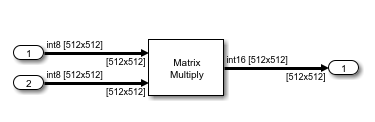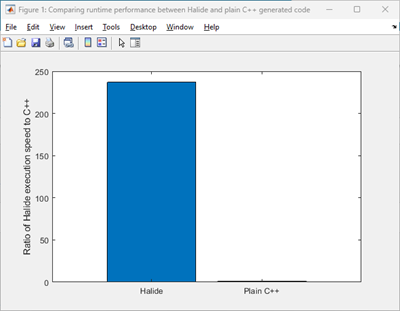## Speed Up Generated Code Execution with Halide Code

Signal processing applications, including applications in deep learning, image processing, and other related fields, often involve computationally intensive tasks that require processing of multidimensional arrays within nested `for`-loops. Performing computations based on nested `for`-loops with multidimensional arrays often introduces performance bottlenecks, hindering the overall efficiency of the operations. To overcome these challenges, you can employ a domain-specific language such as Halide to improve the performance of array computations. Halide is an open-source, domain-specific language designed to optimize algorithms involving multidimensional arrays that is integrated into C++. You can generate Halide code from certain Simulink® blocks if you have an Embedded Coder® license.

The Halide language adopts a functional programming style to describe algorithms, free of traditional control flow constructs such as `for`-loops. Unlike languages like MATLAB® and C++, where `for`-loops dictate the element computation order in an array, Halide distinguishes the algorithm description for array computation from the computation order, referred to as the schedule. This separation also facilitates experimentation with various scheduling techniques to optimize code for different hardware architectures.

Halide is well-suited for algorithms operating on multidimensional arrays, often used in image and signal processing tasks. By leveraging the Halide automated schedulers during the code generation process, the code generator produces highly efficient code that can significantly increase the execution speed of the generated code. For more information about Halide programming, see Halide.

### Generate Halide Code

You can generate Halide code for these blocks in a Simulink model:

To generate Halide code:

1. Open the Embedded Coder app.

2. Click Settings > Code Generation. Set the Generate Halide code parameter. To enable this parameter, check for the dependencies.

3. Build the model.

Note

If there are no opportunities for Halide code generation, Embedded Coder will generate plain C++ code.

### Compare Generated Halide Code to Plain C++ Code

Matrix multiplication is a crucial operation in numerous applications. This example uses the model `MatrixMultiply` that has a Matrix Multiply block. The Matrix Multiply block has input signals with dimension sizes `512` and data type `int8`.The generated plain C++ code for this model is:

``` void MatrixMultiply::step() { int32_T i; int32_T i_0; int32_T i_1; int16_T Out1;```

``` for (i_0 = 0; i_0 < 512; i_0++) { for (i = 0; i < 512; i++) { Out1 = 0; for (i_1 = 0; i_1 < 512; i_1++) { Out1 = static_cast<int16_T>(MatrixMultiply_U.Inport[(i_1 << 9) + i] * MatrixMultiply_U.Inport1[(i_0 << 9) + i_1] + Out1); } MatrixMultiply_Y.Out1[i + (i_0 << 9)] = Out1; } } }```

Halide Code Generation

To generate Halide code, open the Configuration Parameters dialog box and select the Generate Halide code parameter.

The generated Halide code has a Halide Generator class:

` class MatrixMu_matmul_out1_fcn_halide_generator : public Halide::Generator <MatrixMu_matmul_out1_fcn_halide_generator> {`
``` public: Input<Buffer<int8_t>> A1{"A1", 2}; Input<Buffer<int8_t>> B1{"B1", 2}; Output<Buffer<int16_t>> matmul_out1_fcn{"matmul_out1_fcn", 2};```
``` void generate() { RDom r(0, 512); matmul_out1(d1, d2) = sum(cast<int16_t>(A1(d1, r))*cast<int16_t>(B1(r, d2))); matmul_out1_fcn(d1, d2) = matmul_out1(d1, d2); }```
` void schedule() {`
``` if(using_autoscheduler()) { A1.dim(1).set_estimate(0, 512); A1.dim(0).set_estimate(0, 512); B1.dim(1).set_estimate(0, 512); B1.dim(0).set_estimate(0, 512); matmul_out1_fcn.set_estimate(d1, 0, 512).set_estimate(d2, 0, 512); } else { // Default schedule } }```
``` private: Var d1{"d1"}; Var d2{"d2"}; Func matmul_out1{"matmul_out1"}; };```

The arrays are converted to intermediate buffers to work with the complied Halide library.

``` void MatrixMultiply::MatrixMu_matmul_out1_fc_wrapper(const int8_T A1, const int8_T B1, int16_T matmul_out1) { halide_buffer_t u0; halide_buffer_t u1; halide_buffer_t y; int32_T u_size0; u_size0 = 512; u_size0 = 512; u0 = matlabArrayToHalideBuffer(&A1, &u_size0[0U], 2); u1 = matlabArrayToHalideBuffer(&B1, &u_size0[0U], 2); y = matlabArrayToHalideBuffer(&matmul_out1, &u_size0[0U], 2); MatrixMu_matmul_out1_fcn_halide_pipeline(&u0, &u1, &y); deallocateHalideBuffer(&u0); deallocateHalideBuffer(&u1); deallocateHalideBuffer(&y); }```
``` void MatrixMultiply::step() { MatrixMu_matmul_out1_fc_wrapper(&MatrixMultiply_U.Inport, &MatrixMultiply_U.Inport1, MatrixMultiply_Y.Out1); }```

### Compare Code Execution Times

You can run a software-in-the-loop (SIL) simulation to calculate the execution times of the generated Halide code and the plain C++ code for the `MatrixMultiply` model. In this section, you will run SIL simulation programmatically and compare the execution times of your generated code. This will help you to decide whether to choose Halide over plain C++ code.

1. Configure the model to generate plain C++ code.

```model = "MatrixMultiply"; load_system(model); set_param(bdroot,"HalideCodeGeneration",0);```

2. Configure the model to generate a workspace variable to save execution time measurements.

```set_param(model,"CodeExecutionProfiling","on"); set_param(model,"CodeProfilingInstrumentation","off"); set_param(model,"CodeProfilingSaveOptions","AllData");```

3. Run the SIL model simulation.

`out_sil1 = sim(model,"SimulationMode","software-in-the-loop (SIL)");`

4. Use the method `Sections` to extract the code execution time.

```nonhalideSection = out_sil1.executionProfile.Sections(2); nonhalideaverageTime = double(nonhalideSection.TotalExecutionTimeInTicks)/double(nonhalideSection.NumCalls); ```

5. Configure the model to generate Halide code and run the simulation again.

```set_param(bdroot,"HalideCodeGeneration",1); out_sil2 = sim(model,"SimulationMode","software-in-the-loop (SIL)"); halideSection = out_sil2.executionProfile.Sections(2); halideaverageTime = double(halideSection.TotalExecutionTimeInTicks)/double(halideSection.NumCalls); ```

6. Compare the difference in code execution speed.

```speedup = nonhalideaverageTime/halideaverageTime; fprintf("Speedup factor of Halide code compared to plain C++ = %f\n", speedup) ```
`Speedup factor of Halide code compared to plain C++ = 237.015285`
```cgLabels = categorical({'Plain C++','Halide'}); runtimeTicks = [1, speedup]; bar(cgLabels, runtimeTicks); ylabel("Ratio of Halide execution speed to C++"); title("Comparing runtime performance between Halide and plain C++ generated code");```The simulation was run on AMD EPYC™ 74F3 24-Core Processor @ 3.19 GHz test system. For the `MatrixMultiply` model, Halide code is approximately 237 times faster than the plain C++ code.

Note

Halide code significantly improves the execution speed for operations involving contiguous large multidimensional arrays. It might not perform well for smaller arrays. Based on the dimension size of the array, Embedded Coder decides whether to generate Halide code or plain C++ code for a model.

### Limitations

• Halide code generation is not supported for:

• Fixed-point and half-precision data types

• Complex data type

• Halide code will not be generated if the dimension size of the array is below a certain threshold value.

• Halide code may not be generated for models that have a referenced model.

• Static code metrics report generation is not supported.

• Halide code generation is supported only when the Hardware Board parameter is set to `none` and assumes that the host computer is the production hardware.